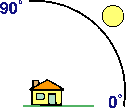## Overview

A color 3D representation of the terrain is created by merging the images from the elevation-coded and hillshade methods. This function uses the altitude and azimuth properties to specify the sun's position.

## Notes

### Unit conversion

If the units for the z (elevation) units are the same as the x,y (linear) units, then the z conversion factor is 1. If your dataset is using a projected coordinate system, your scaling is set to None, and your elevation and linear units are different, then you will need to define a z conversion factor to account for the difference.

To convert from feet to meters or vice versa, see the table below. For example, if your DEM's elevation units are feet and your mosaic dataset's units are meters, you would use a value of 0.3048 to convert your elevation units from feet to meters (1 foot = 0.3048 meters).

Conversion typeConversion factor

Feet to meters

0.3048

Meters to feet

3.28084

If your data is using a geographic coordinate system (such as a DTED in GCS_WGS 84), where the linear units are in degrees and your elevation is in meters, use a conversion factor of 1, and the system will automatically convert your linear degrees to meters. If your elevation units are not in meters, use the Unit Conversion function to convert your elevation to meters before using this function.

##### Note:

When your scaling is set to Adjusted, you can use the Z Factor to convert z units to meters, and ArcGIS will automatically adjust the latitude and longitude to meters.

### Vertical exaggeration

To apply vertical exaggeration, you must multiply the conversion factor by the exaggeration factor. For example, if both elevation and dataset coordinates are meters and you want to exaggerate by a multiple of 10, the scaling factor would be the unit conversion factor (1.0) multiplied by the vertical exaggeration factor (10.0)—which would be a Z Factor of 10. If the elevation units are meters and the dataset is geographic (degrees), you would multiply the unit conversion factor (1.0) by the exaggeration factor (10.0)—which would be a Z Factor of 10.

## Parameters

The inputs for this function are the following:

ParameterDescription

Raster

The input elevation dataset.

Color Scheme Type

Specify whether a color ramp or a color map will be used to display the shaded relief.

• Color Ramp—When you choose Color Ramp, you will be prompted to select an appropriate color ramp.
• Colormap—When you choose Colormap, you will be prompted to specify the color map file to use.

Controls the illumination source for the hillshade.

• Traditional—Calculates hillshade from a single illumination direction. You can set the Azimuth and Altitude options to control the location of the light source.
• Multidirectional—Combines light from multiple sources to represent an enhanced visualization of the terrain.

Azimuth

Azimuth is the sun's relative position along the horizon (in degrees). This position is indicated by the angle of the sun measured clockwise from due north. An azimuth of 0 degrees indicates north, east is 90 degrees, south is 180 degrees, and west is 270 degrees.

Altitude

Altitude is the sun's angle of elevation above the horizon and ranges from 0 to 90 degrees. A value of 0 degrees indicates that the sun is on the horizon, that is, on the same horizontal plane as the frame of reference. A value of 90 degrees indicates that the sun is directly overhead. The default is 45 degrees above the horizon.Scaling

The shaded result is scaled dynamically by adjusting the z-factor using one of two options:

• None—No scaling is applied. This is ideal for a single raster dataset covering a local area. This is not recommended for worldwide datasets with large variations in elevation or multiscale maps, as it will produce terrain relief with little variation at small scales.
• Adjusted—A nonlinear adjustment is applied using the default Pixel Size Power and Pixel Size Factor values, which accommodate a wide variety of altitude changes (scale) as the viewer zooms in and out. The Adjusted option is recommended when using a worldwide dataset.
The default is None.

Z Factor

The z-factor is a scaling factor used to convert the elevation values for two purposes:

• To convert the elevation units (such as meters or feet) to the horizontal coordinate units of the dataset, which may be feet, meters, or degrees
• To add vertical exaggeration for visual effect

The shaded result is scaled dynamically by adjusting the z-factor using one of two options:

• None—No scaling is applied. This is ideal for a single raster dataset covering a local area. This is not recommended for worldwide datasets with large variations in elevation or multiscale maps, as it will produce terrain relief with little variation at small scales.
• Adjusted—A nonlinear adjustment is applied using the default Pixel Size Power and Pixel Size Factor values, which accommodate a wide variety of altitude changes (scale) as the viewer zooms in and out. The Adjusted option is recommended when using a worldwide dataset.
The default is None.

Disable default edge pixel interpolation

Specify whether the default edge pixel interpolation with be disabled.

• Unchecked—Bilinear resampling will be applied uniformly to resample the shaded relief. This is the default.
• Checked—Bilinear resampling will be used within the shaded relief, except along the edges of the rasters or next to pixels of NoData. These pixels will be populated with NoData, since it will reduce any sharp edge effects that may occur.

Using this option will avoid any resampling artifacts that may occur along the edges of a raster. The output pixels along the edge of a raster or beside pixels without a value will be populated with NoData; therefore, it is recommended that this option be used only when there are other rasters with overlapping pixels available. When overlapping pixels are available, these areas of NoData will display the overlapping pixel values instead of being blank.